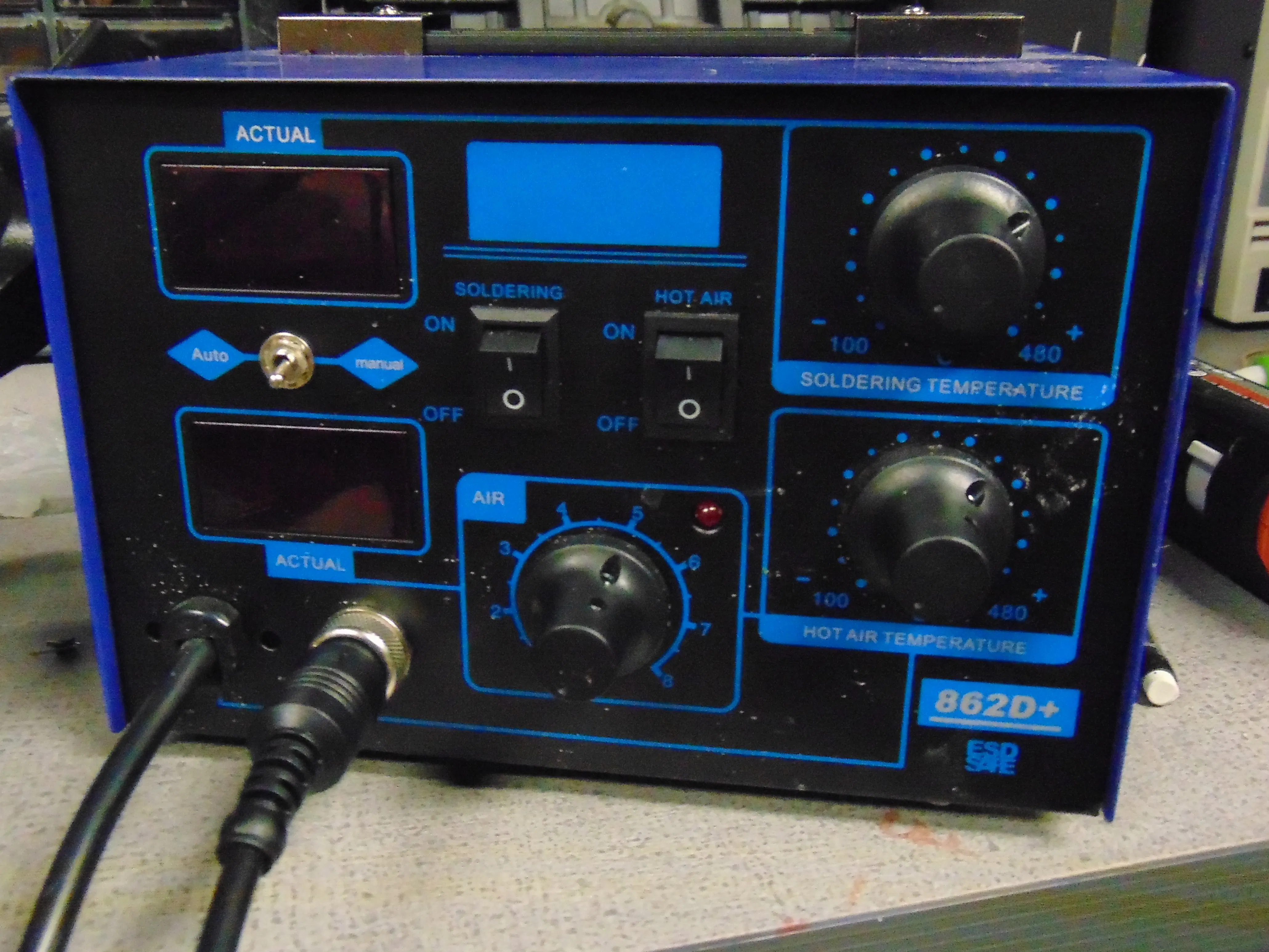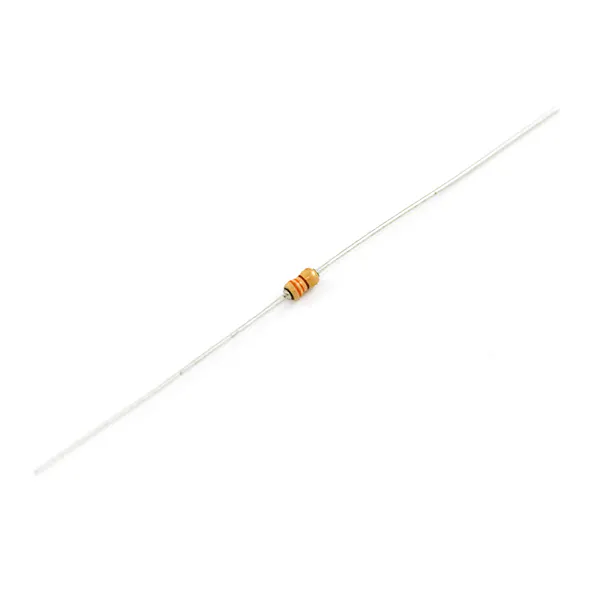Project tutorialDIY Soldering Iron Control for 862D+ © LGPL

Did your somewhat expensive (\$50) soldering iron just die? Well then fix it with another one!

• 2,884 views
• 17 respects

Components and suppliesArduino Nano R3
×1
 Normal Flyback Diode.
×1
 irf540n n-channel mosfet
×1
 5v linear regulator
×1Resistor 330 ohm
×1
×2
 .96 inch Oled
×1

Necessary tools and machinesSoldering iron (generic)
 8mm drill bit /w drill

Update (10/21/2019): Major update to code. Also take a read below. A bit of it has changed.

My 862D+ soldering iron broke, so instead of buying a new one I took a second iron and fixed it. The hardest part is that there was no solution elsewhere online. The PCB was broken because after replacing the iron it still said S-E or sensor error.

So I decided to create my own controller circuit, and stuff it inside. It required a second transformer but being cheap, there was plenty of room inside.

It may not look good, but it works like a charm. As a bonus, I removed the soldering iron power button, and added it between 5v and the arduino.

Onto the build tips:

All parts can be bought now. LCSC is a bulk electronics site. Shipping is expensive to America, but the components are ridiculously cheap.

EX mosfet on Amazon \$6 but mosfet on LCSC 32 cents. However things like resistors must be bought in bulk. Still, 50 resistors are literally .84 cents.

MAKE SURE TO SHIELD LONG DATA WIRES with grounded aluminum foil or something else. The 12c screen wire for example.

In the code:

1023 coldest 0 lava. Change the settemp to you desire. Somewhere around 600 typically.

The soldering iron controller can detect whether your iron is connected or broken. If the soldering iron is disconnected, the arduino will zero the analog input. If the soldering iron used to heat up properly, but does not now: disconnect the iron, wait until it says plug iron in, then you are good to go. (This has been an issue for me)

Now you know the basics of your iron. This can be a cheap alternative to buying a quality soldering iron. While there are higher quality irons, this one is very cheap and diy.

Code

Control CodeC/C++
Paste this into the arduino and upload. This only works with the circuit provided
int output = 0;
int temp = 0;
int settemp = 630;
String inputString = "";         // a string to hold incoming data
boolean rap = false;
boolean stringComplete = false;  // whether the string is complete
int t = 0;

void setup() {
Serial.begin(9600);
inputString.reserve(200);
pinMode(6, OUTPUT);
digitalWrite(6, LOW);
}

void loop() {
serialEvent();
if (stringComplete) {
Serial.println(inputString);
t = inputString.toInt();
//settemp = t //sets the set temp to the serial input
inputString = "";
stringComplete = false;
}
rapid();
pid();
}

void rapid() { //Controls the soldering iron by rapidly heating it up in the beginning.
if (rap == false) {
digitalWrite(6, HIGH);
do {
Serial.print("rapid");
Serial.println(temp);
} while (temp - settemp > 10);
digitalWrite(6, LOW);
rap = true;
}
}

void pid() { //Controls the soldering with slow active power heating.
output = temp - settemp;
if (output < 0) {
output = 0;
}
if (output > 255) {
output = 255;
}
Serial.print("pid");
Serial.print(temp);
Serial.print(", ");
Serial.println(output);
analogWrite(6, output);
}

void serialEvent() {  //Serial communication that can be used to update settemp
while (Serial.available()) {
char inChar = (char)Serial.read();
inputString += inChar;
if (inChar == '\n') {
stringComplete = true;
}
}
}
The CodeC/C++
Paste into editor and upload. Only works with designed schematic.
#include <Wire.h>

int settemp = 590; //manual set variables

int output = 0;
int temp = 0;
String inputString = "";
boolean rap = false;
boolean stringComplete = false;
int t = 0;
int mappedpower = 0;
byte powermultiply = 0;
long counter = 0;
int temp2 = 0;
byte outputoffset = 0;
byte scaleread = 0;

void setup() {
Serial.begin(9600);
pinMode(12, OUTPUT);
digitalWrite(12, LOW);
display.clearDisplay();
display.display();
rapid();
}

void loop() {
pid();
display.clearDisplay();
updatebar();
updatetext();
display.display();
}

void rapid() { //Controls the soldering iron by rapidly heating it up in the beginning.
if (rap == false) {
digitalWrite(12, HIGH);
output = 255;
do {
Serial.print("rapid");
Serial.println(temp);
display.clearDisplay();
updatebar();
updatetext();
display.display();
} while (temp - settemp > 1);
digitalWrite(12, LOW);
rap = true;
}
}

void pid() { //Controls the soldering with slow active power heating.
temp = analogRead(0);  //Temp averager
temp2 = temp;
delay(50);
temp = temp + temp2;
temp = temp / 2;

zeroscale; //activate no soldering iron checker
temp = temp + outputoffset;

output = temp - settemp;
output = output + powermultiply;
output = output * 4;

if (output < 0) {
output = 0;
}
if (output > 255) {
output = 255;
}
counter = counter + 1;
if (counter > 10) {
counter = 0;
if (temp > settemp + 3) {
powermultiply = powermultiply + 1;
}
if (temp < settemp - 3 && powermultiply > 0) {
powermultiply = powermultiply - 1;
}
}
Serial.print(powermultiply);
Serial.print(", ");
Serial.print(temp);
Serial.print(", ");
Serial.println(output);
analogWrite(12, output);
}

void updatebar() { //Updates Powerbar on OLED display
mappedpower = map(output, 0, 255, 0, 127) - 10;
display.fillRect(0, 0, mappedpower, 8, WHITE);
display.fillRoundRect(mappedpower - 5, 0, 20, 8, 3, WHITE);
}

void updatetext() {//Update text on Oled display
display.setCursor(0, 9);
display.setTextColor(WHITE);
display.setTextSize(1);
display.print("Set Temp: ");
display.print(settemp);
display.setCursor(0, 20);
display.print("Temp: ");
display.print(temp);
}

void zeroscale() { //If soldering iron is unplugged, adjust the input pin value to zero it out
if (output < 200) {
display.clearDisplay();

display.setCursor(0, 9);
display.setTextColor(WHITE);
display.setTextSize(1);
display.print("No soldering iron, or defective");
display.setCursor(0, 15);
display.print("iron.");
display.setCursor(0, 20);
display.print("Testing in 5 seconds.");

display.display();
delay(5000);

if (output < 200) {
outputoffset = temp;
}
display.clearDisplay();

display.setCursor(0, 9);
display.setTextColor(WHITE);
display.setTextSize(1);
display.print("Output offset set.");
display.setCursor(0, 20);
display.print("Please plug in the iron.");

display.display();
do {
} while (scaleread < 200);

}
}
Code Updated 10/21/2019C/C++
Paste in IDE and upload. Only works with shematic.
#include <Wire.h>

int settemp = 590; //manual set variables

int output = 0;
int temp = 0;
String inputString = "";
boolean rap = false;
boolean stringComplete = false;
int t = 0;
int mappedpower = 0;
byte powermultiply = 0;
long counter = 0;
int temp2 = 0;
byte outputoffset = 0;
byte scaleread = 0;

void setup() {
Serial.begin(9600);
pinMode(12, OUTPUT);
digitalWrite(12, LOW);
display.clearDisplay();
display.display();
rapid();
}

void loop() {
pid();
display.clearDisplay();
updatebar();
updatetext();
display.display();
}

void rapid() { //Controls the soldering iron by rapidly heating it up in the beginning.
if (rap == false) {
digitalWrite(12, HIGH);
output = 255;
do {
Serial.print("rapid");
Serial.println(temp);
display.clearDisplay();
updatebar();
updatetext();
display.display();
} while (temp - settemp > 1);
digitalWrite(12, LOW);
rap = true;
}
}

void pid() { //Controls the soldering with slow active power heating.
temp = analogRead(0);  //Temp averager
temp2 = temp;
delay(50);
temp = temp + temp2;
temp = temp / 2;

zeroscale(); //activate no soldering iron checker
temp = temp + outputoffset;

output = temp - settemp;
output = output + powermultiply;
output = output * 4;

if (output < 0) {
output = 0;
}
if (output > 255) {
output = 255;
}
counter = counter + 1;
if (counter > 10) {
counter = 0;
if (temp > settemp + 3) {
powermultiply = powermultiply + 1;
}
if (temp < settemp - 3 && powermultiply > 0) {
powermultiply = powermultiply - 1;
}
}
Serial.print(powermultiply);
Serial.print(", ");
Serial.print(temp);
Serial.print(", ");
Serial.println(output);
analogWrite(12, output);
}

void updatebar() { //Updates Powerbar on OLED display
mappedpower = map(output, 0, 255, 0, 127) - 10;
display.fillRect(0, 0, mappedpower, 8, WHITE);
display.fillRoundRect(mappedpower - 5, 0, 20, 8, 3, WHITE);
}

void updatetext() {//Update text on Oled display
display.setCursor(0, 9);
display.setTextColor(WHITE);
display.setTextSize(1);
display.print("Set Temp: ");
display.print(settemp);
display.setCursor(0, 20);
display.print("Temp: ");
display.print(temp);
}

void zeroscale() { //If soldering iron is unplugged, adjust the input pin value to zero it out
if (temp < 200) {
display.clearDisplay();

display.setCursor(0, 20);
display.setTextColor(WHITE);
display.setTextSize(1);
display.print("No soldering iron.");
display.display();
delay(10000);

display.setCursor(0, 0);
display.print("Testing in 5 seconds.");

display.display();
delay(5000);

if (temp < 200) {
outputoffset = temp;
}
display.clearDisplay();

display.setCursor(0, 20);
display.setTextColor(WHITE);
display.setTextSize(1);
display.print("Output offset set.");
display.setCursor(0, 0);
display.print("Connect the iron.");

display.display();
do {
} while (scaleread < 200);

}
}

Schematics

Use this schematic to create a circuit for the ironSoldering Iron Controller for Hakko 907

Project tutorial by Alexander

• 34,555 views
• 71 respects

Soldering Iron Controller for Hakko 907 v.2

Project tutorial by Alexander

• 29,777 views
• 69 respects

Simple Arduino Soldering Station

Project showcase by lazyvlad

• 17,429 views
• 40 respects

DIY SMD Rework Station

Project tutorial by Alexander and Maker B R

• 10,506 views
• 48 respects

How to Make a Gesture Control Robot at Home

Project tutorial by Shubham Shinganapure

• 3,552 views